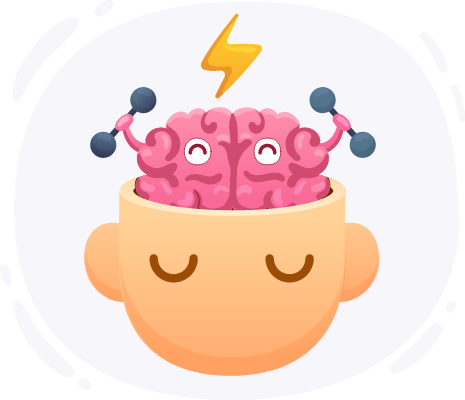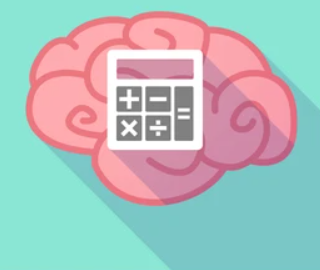Online Math Center

# How To Develop And Improve Your Student’s Mental Math Skills

Mental math is a skill set that enables children to do math in their head without using calculators, pen or paper. Mental math involves more than just memorizing the multiplication table by heart or answering a math problem fast.

Mental math is about using cognitive strategies to calculate mentally. Children who are good at mental math improve their computational fluency or number sense. It also makes them develop efficiency, accuracy and flexibility, which are important educational qualities.

In this article we will examine a few mental math skills and their benefits. We will also look at ways to improve your student’s mental math skills.

## What Are Mental Math Skills?Mental math is the ability to do math calculations in your mind quickly and efficiently. Mental math skills include remembering math facts, rounding numbers and estimating calculations.

For example, what is 47+65? It is easier to do this mentally when you break down the numbers into digit values i.e. 40 + 60= 100 and 7+5= 12. Add the totals and you will get 112 as an answer.

Students with strong mental math skills have a better working memory and are able to retain math facts fast. Mental math skills differ from one person to the other; some students are better than others. When children struggle with mental math that doesn’t mean they are not smart. Trouble doing mental math could mean that your student needs more time to practice math.

## Benefits Of Mental Math

Mental math can be of great use anywhere, not only in a math class. Mental math is of great use when shopping, paying bills and in everyday life. For example, when shopping mental math can help you calculate the price of an item, it lets you know how much cash to give the cashier and calculate your change.

Practicing mental math frequently isn’t an easy feat; it requires regular studying. Students who are adept at mental math understand math concepts better and can practice speed math. They also have a deeper understanding of the subject beyond memorizing the time table. Mental math skills are worth developing because they remain with students for life no matter what profession they choose to take.

To enable children to practice mental math teachers need to make them solve math problems mentally. For starters, you can conduct a basic exercise with addition, subtraction or multiplication and then move on to more advanced levels of math.

## Strategies To Improve Mental Math

Teachers can use everyday life skills to teach students mental math. For example, doubling down a recipe in a cooking class, counting change in a piggy bank and scoring a game of scrabble are all creative strategies. They involve using knowledge of basic math operations to work out an answer.

There are also other strategies teachers could use to enhance a student’s mental math. Let us explore them.

1. Playing Math Games

Math games enable students to build recall and multiplication skills to improve their mental math. Games like math facts bingo for example, allows teachers to create bingo cards with answers to different questions. Teachers then hand a card to each student and instead of calling out the numbers state the equation such as 8 x 7. After determining the answer is 56 students can check off the number on their cards.

Stand up and sit down is another interesting game. Pick a number and share it with your students, then read the equations out loud. Sitting in a circle each student must stand if the answer matches the number you picked. If they stand incorrectly or remain seated, eliminate them until one student remains. A few rounds of these games can improve a student’s math fluency.

2. Use Math Apps And Websites

Math apps and websites are a fun alternative to math drills and worksheets. Math websites are a creative alternative prompting students to answer math questions and build their mental math skills. Prodigy Math  for example, is aligned with most math curriculums and is designed to differentiate content and give adaptive feedback to students. Math is fun has a range of creative content for young ones covering basic math concepts with games and puzzles.3. Round Up Numbers

Students can learn to roundup numbers when they are confronted with difficult questions. For example, students can use existing rounding and fact fluency when multiplying by 9, 99,999 or any other number that follows that pattern.

Ask your students to round up the 9 to 10 then after you solve the equation teach them to subtract the number they multiplied by 10 from their answer. For example, 67x 9 will give you the same answer as 67 x 10 – 67. Even though more steps are involved, altering the equation in this way is faster and easier to complete mentally.

3. Use Multiplication Tricks

Memorizing the multiplication table by heart is challenging for most elementary school children, a few tricks can make it easier. You can use the following strategies:

• Any number times 1 remains the same.

• Any number times 10 has a zero added to its end.

• Any number up to 9 times 11 is just the same digit repeated twice (e.g., 99).

### OMC Sharpens Students Mental Math

The Online Math Center tutors use a range of math learning strategies along with creative activities to refine your child’s mental math skills.

## Step One

It only takes two steps to schedule a free lesson with an OMC representative.

To get started, please tell us who you are:

## Dear Parent!

Thank you for placing your trust in us to educate your child!

Congratulations on joining the Online Math Center!
See you in class.

## Thank you!

Our manager will be in contact with you shortly.

Please check your inbox for more information. If there is no message from us, please check your email’s “Spam” folder.

## Step Two

Let us know how to contact you. One of our representatives will get back to you shortly.

## Step Two

Awesome! We need to get in touch with your parent or guardian for further discussion. Please check in with them before filling out the form below with their information.

## Step One

It only takes two steps to schedule an appointment with an OMC representative.

To get started, please tell us who you are: